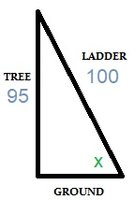# Problems

#### Dr.Peterson

##### Elite Member
These pictures are very hard to read, and a lot seems to have been cut off.

Please be kind to those who are helping, by typing in one problem at a time, together with your work, and telling us where you need help -- for example, what part of the work you are unsure of.

Pictures can be helpful to clarify a problem, but not as the main mode of communication, and they must be made carefully in order to be easily readable.

If you didn't already, be sure to read our submission guidelines, which tell you how to ask a question to get the most out of the site.

#### Otis

##### Senior Member
Hello Carrotchan. In the first exercise (Trent's Tree Trimming), you are correct to draw a right triangle, although I would orient the triangle so that the tree is vertical instead of horizontal. (The tree is not laying on the ground.)

The exercise asks about the unknown angle between the ladder and the ground, so let's not label it 80º because we don't know what it is, yet. We assume the top of the 100-foot ladder meets the top of the 95-foot tree. Here's a sketch. I picked symbol x to represent the unknown angle, and x is measured in degrees.The side opposite angle x is 95, and the hypotenuse is 100. The right-triangle definition of sine is this ratio: Opposite/Hypotenuse. Therefore, we write the equation

sin(x) = 95/100

Have you learned how to find an angle, when you know its sine value? (I would use a scientific calculator's inverse-sine function, for that.)

After you find angle x, then compare it to 80º. If x is greater than 80º, then Trent has violated the safety regulation. If x is not greater than 80º, then Trent has not violated the regulation.#### Otis

##### Senior Member
In the second exercise (fill in the boxes), you filled in the first set of boxes with
$\frac{sin(A)}{sin(B)} = cos(B)$

Let's check that, by applying the right-triangle definitions for sine (Opposite/Hypotenuse) and cosine (Adjacent/Hypotenuse). For the side lengths, we use the standard symbols given in the diagram.
$sin(A)=\frac{a}{c} \quad sin(B)=\frac{b}{c} \quad cos(B)=\frac{a}{c}$

$$\dfrac{\frac{a}{c}}{\frac{b}{c}} = \dfrac{a}{c}$$

$$\dfrac{a}{\cancel{c}} \cdot \dfrac{\cancel{c}}{b} = \dfrac{a}{c}$$

$$\dfrac{a}{b} = \dfrac{a}{c}$$

We get a false result, so the answer is not correct.

Here's a hint: The tangent of an angle may be written as the ratio of the angle's sine to its cosine. We state this fact as an identity -- an equation that's true for all angles x in the domain.
$tan(x) = \frac{sin(x)}{cos(x)}$

You can use this identity, to find a correct answer for the first set of boxes.

In the next set of boxes, you wrote $$sin(B) = tan(B)$$

We know this is not correct because sine (Opposite/Hypotenuse) is different than tangent (Opposite/Adjacent) for all angles other than the trivial case of 0º. Let's show how they differ for angle B, by applying the definition for each side:
$sin(B)=\frac{b}{c} \quad tan(B)=\frac{b}{a}$

Those ratios are not equal. Hint: Write the sine and cosine ratios for each angle A and B. Then you will see which ratios are equal.

In the last set of boxes, you wrote
$tan(A) \cdot tan(B) = 1$

We can check this, also, by using the right-triangle definition for tangent (Opposite/Adjacent).
$tan(A)=\frac{a}{b} \quad tan(B)=\frac{b}{a}$

Yes, your answer is correct because a/b and b/a are reciprocals, and we know the product of two reciprocals is always 1.#### Otis

##### Senior Member
Now that you've seen how to find and check answers using the right-triangle definitions for sine, cosine and tangent, please try the third exercise again (true or false exercises [a] through [e] concerning three similar right triangles). You indicated that xº=yº=zº is true, and that is correct because those are corresponding angles in three similar triangles. Corresponding angles within any similar triangles are equal. There's also a theorem from geometry that tells us parallel lines intersecting a third line (transversal) form certain equal angles.

If you would like more help, please show your work more completely, so that tutors can see what you're doing.

Others may reply, but for now I'm going to wait for your response before continuing. I would like to see whether you understand how to use the right-triangle definitions for sine, cosine and tangent.